# Kodaira dimension

Jump to: navigation, search

A numerical invariant of an algebraic variety, named after K. Kodaira who first pointed out the importance of this invariant in the theory of the classification of algebraic varieties.

Letbe a non-singular algebraic variety and letbe a rational mapping defined by a linear system, where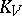is the canonical class of. The Kodaira dimension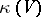ofis defined as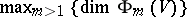. Here, iffor all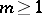, then it is assumed that. The Kodaira dimension is a birational invariant, that is, it does not depend on the representative in the birational equivalence class.

Suppose that the ground field is the field of the complex numbers. Ifis sufficiently large, then one has the estimate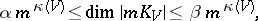where,are certain positive numbers. If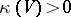, then there exists a surjective morphism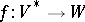of algebraic varieties such that: a)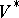is birationally equivalent to; b); and c) for some dense open set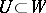, all the fibres,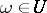, are varieties of parabolic type (i.e. of Kodaira dimension zero).

There is a generalization of the notion of the Kodaira dimension (see ) to the case when in the linear systemthe canonical classis replaced by an arbitrary divisor.